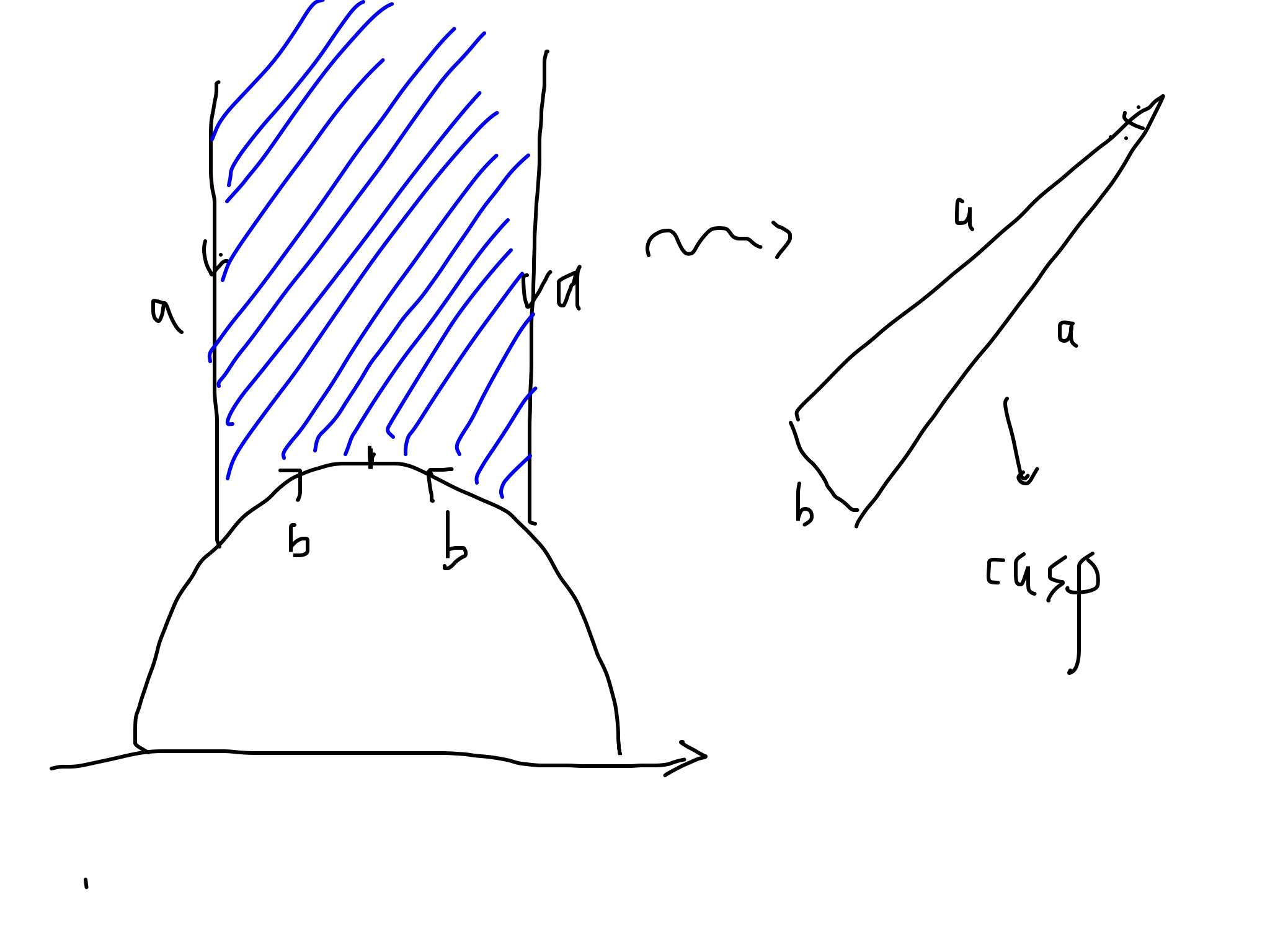# SL_2(Z) and its congruence subgroups

We know we can always do the following thing:$\displaystyle R\ commutative\ ring \longrightarrow \ "general\ linear\ group" \ GL_2(R) \ \ \ \ \ (1)$

Where$\displaystyle GL_2(R):=\{\begin{pmatrix} a & b \\ c & d \end{pmatrix}: det \begin{pmatrix} a & b \\ c & d \end{pmatrix}=R^*, a,b,c,d\in R\} \ \ \ \ \ (2)$

Remark 1 Why it is${R^*}$ but not 1? if it is 1, then the action${R/GL_2(R)}$ distribute is not trasitive on${R}$, i.e. every element in unite group present a connected component

Now we consider the subgroup${SL_2(R)\subset GL_2(R)}$.$\displaystyle SL_2(R)=\{\begin{pmatrix} a & b\\ c & d \end{pmatrix}: det \begin{pmatrix} a & b\\ c & d \end{pmatrix}=1, a,b,c,d\in R \} \ \ \ \ \ (3)$

We are most interested in the case${R={\mathbb Z}, {\mathbb Z}/n{\mathbb Z}}$. So how to investigate${SL_2({\mathbb R})}$? We can look at the action of it on something, for particular, we look at the action of it on Riemann sphere, i.e.${ \hat {\mathbb C}/({\mathbb R})}$ given by fraction linear map:$\displaystyle g(z):=\frac{az+b}{cz+d}, g(\infty)=\frac{a}{c} \ \ \ \ \ (4)$

Remark 2 What is fraction linear map? This action carry much more information than the action on vector, thanks for the exist of multiplication in${{\mathbb C}}$ and the algebraic primitive theorem. Due to I always looks the fraction linear map as something induce by the permutation of the roots of polynomial of degree 2, this is true at least for fix points, and could natural extension. So how about the higher dimension generate? consider the transform of${k-1}$ tuples induce by polynomial with degree${k}$?

Remark 3

1.${SL_2({\mathbb R})/\pm I:=PSL_2({\mathbb R})}$, then${PSL_2({\mathbb R}) }$ action faithful on${\hat C}$, i.e. except identity, every action is nontrivial. This is easy to be proved, observed,$\displaystyle \frac{az+b}{cz+d}=z,\forall z\in \hat{\mathbb C}\Longrightarrow \begin{pmatrix} a & b\\ c & d \end{pmatrix}=\begin{pmatrix} 1 & 0\\ 0&1 \end{pmatrix} or \begin{pmatrix} -1&0\\ 0&-1 \end{pmatrix} \ \ \ \ \ (5)$

2. Up half plane${H}$ is invariant under the action of${PSL_2({\mathbb R})}$, i.e.${\forall g\in PSL_2({\mathbb R})}$,${gH=H}$. The proof is following,
3.$\displaystyle \begin{array}{rcl} Im(\frac{az+b}{cz+d}) & = & Im(\frac{(az+b)(c\bar z+d)}{|cz+d|^2})\\ & = & Im(\frac{ac|z|^2+bc\bar z+adz+bd}{|cz+d|^2})\\ & > & 0,\ due\ to\ ad=bc+1. \end{array}$

Now we focus on${SL_2({\mathbb Z})}$ or the same,${PSL({\mathbb Z})}$. All the argument for${SL_2(R)}$ make sense for$\displaystyle \Gamma:= SL_2({\mathbb Z}), \bar \Gamma:=SL_2({\mathbb Z})/\pm I \ \ \ \ \ (6)$

Fix${N\in {\mathbb N}}$, define,$\displaystyle \Gamma(N):=\{\begin{pmatrix} a &b\\ c&d \end{pmatrix}, a,d \equiv 1(mod N), b,c\equiv 0(mod N)\} \ \ \ \ \ (7)$

Then${\Gamma(N)}$ is the kernel of map${SL_2({\mathbb Z})\rightarrow SL_2({\mathbb Z}/n{\mathbb Z})}$, i.e. we have short exact sequences,$\displaystyle 0\longrightarrow \Gamma(N)\longrightarrow \Gamma\longrightarrow SL_2({\mathbb Z}/n{\mathbb Z})\longrightarrow 0 \ \ \ \ \ (8)$

Remark 4 The relationship of${\Gamma(N)\subset \Gamma}$ is just like${N{\mathbb Z}+1\subset {\mathbb Z}}$.

Definition 1 (Congruence group) A subgroup of${\Gamma}$ is called a congruence group iff${\exists n\in {\mathbb N}}$,${\Gamma(N)\subset G}$.

Example 1 We give two examples of congruence subgroups here.

1.$\displaystyle \Gamma_1(N)=\{\begin{pmatrix} 1& *\\ 0 &1 \end{pmatrix} mod N\} \ \ \ \ \ (9)$

2.$\displaystyle \Gamma_0(N)=\{\begin{pmatrix} * & *\\ 0 & * \end{pmatrix}mod N\} \ \ \ \ \ (10)$

Definition 2 (Fundamental domain)$\displaystyle F=\{z\in H:-\frac{1}{2}\leq Re(z)\leq \frac{1}{2}\ and |z|\geq 1\} \ \ \ \ \ (11)$

Now here is a theorem charistization the fundamental domain.Theorem 3 This domain${F}$ is a fundamental domain of${\hat {\mathbb H}/({\mathbb R})}$

Proof: Ths key point is${SL_2({\mathbb Z})}$ have two generators,

1.${\tau_a: z\rightarrow z+a, \forall a\in {\mathbb Z}}$.
2.${s: z\rightarrow \frac{1}{z}}$.

Thanks to this two generator exactly divide the action of${\Gamma}$ on${H}$ into a lots of scales, then${\Omega}$ is a fundamental domain is a easy corollary.$\Box$

Remark 5 This is not rigorous,${H}$ need be replace by${\hat H}$, but this is very natural to get a modification to a right one.

Remark 6${z_1,z_2\in \partial F}$ are${\Gamma}$ equivalent iff${Re(z)=\pm \frac{1}{2}}$ and${z_2=z_1\pm 1}$ or if${z_1}$ on the unit circle and${z_2=-\frac{1}{z_1}}$

Remark 7 If${z\in F}$, then${\Gamma_z=\pm I}$ expect in the following three case:

1.${\Gamma=\pm \{\tau,s\}}$ if${z=i}$.
2.${\Gamma=\pm\{ I,s\tau, (s\tau)^2\}}$ if${z=w=-\frac{1}{2}+\frac{\sqrt{-3}}{2}}$.
3.${\Gamma=\pm\{I,\tau s, (\tau s)^2\}}$ if${z=-\bar w=\frac{1}{2}+\frac{\sqrt{-3}}{2}}$.

Where${\tau=\tau_1}$.

Remark 8 The group${\bar \Gamma=SL_2({\mathbb Z})/\pm I}$ is generated by the two elements${s}$,${\tau}$. In other word, any fraction linear transform is a “word” induce by${s,\tau,s^{-1}.\tau^{-1}}$. But not free group, we have relationship${s^2=-I,(s\tau)^3=-I}$.The natural function space on${F}$ is the memorphic function, under the map:${H\rightarrow D-\{0\}}$, it has a${q}$-expension,$\displaystyle f(q)=\sum_{k\in {\mathbb Z}}a_kq^k \ \ \ \ \ (12)$

And there are only finite many negative${k}$ such that${a_k\neq 0}$.